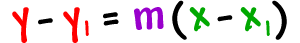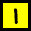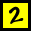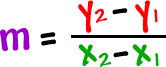In the last lesson, I showed you how to get the equation of a line given a point and a slope using the formulaAnytime we need to get the equation of a line, we need two thingsa pointa slope

ALWAYS!

So, what do we do if we are just given two points and no slope?

No problem -- we'll just use the two points to pop the slope using this guy:Check it out:

Let's find the equation of the line that passes through the pointsThis one's a two-stepper...

STEP 1:  Find the slope